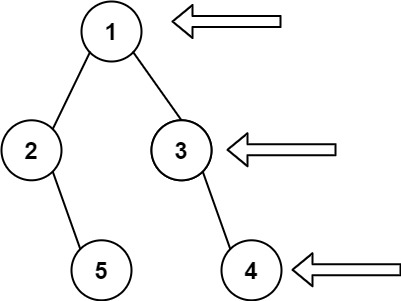# Binary Tree Right Side View

## Binary Tree Right Side View

Given the `root` of a binary tree, imagine yourself standing on the right side of it, return the values of the nodes you can see ordered from top to bottom.

Example 1:```Input: root = [1,2,3,null,5,null,4]
Output: [1,3,4]
```

Example 2:

```Input: root = [1,null,3]
Output: [1,3]
```

Example 3:

```Input: root = []
Output: []
```

Constraints:

• The number of nodes in the tree is in the range `[0, 100]`.
• `-100 <= Node.val <= 100`

### Solution to to the above question

class Solution {
public:vector<int>v;
vector<int>ans;

vector<intrightSideView(TreeNode* root) {
if(root==NULL){
return ans;
}

queue<TreeNode*>q;
q.push(root);
q.push(NULL);

while(!q.empty()){
TreeNode* temp=q.front();
q.pop();
if(temp==NULL){
v.push_back(ans);
ans.clear();
if(!q.empty()){
q.push(NULL);
}
}
else{
ans.push_back(temp->val);
if(temp->left!=NULL){
q.push(temp->left);
}
if(temp->right!=NULL){
q.push(temp->right);
}
}

ans.clear();
}

return v;
}
};